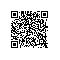# c++ 由类型转换引起的指针偏移

1. 由虚函数引起的指针偏移

class X {
int i;
};
class Y : public X {
int j;
public:
virtual void vf();
};

X的内存布局很简单，由于它没有虚函数，所以没有vptr,只有一个int类型的变量i;

 vptr int i int j

Y y;
X* pX = &y;

X* pX = (X*)((char*)&y + sizeof(vptr))

2. 由多重继承引起的指针偏移

class A {
int i;
};
class B {
int j;
};
class C : public A, public B {
int k
};

C的内存布局可能像这样：
 int i int j int k

C c;
B* pb = &c;

B* pb = (B*)((char*)&c + sizeof(A));

B* pb = (pc) ? (B*)((char*)pc + sizeof(A)) : 0; //假设pc是一个指向C的指针

3.由虚继承引起的指针偏移使用钉钉扫一扫加入圈子
+ 订阅